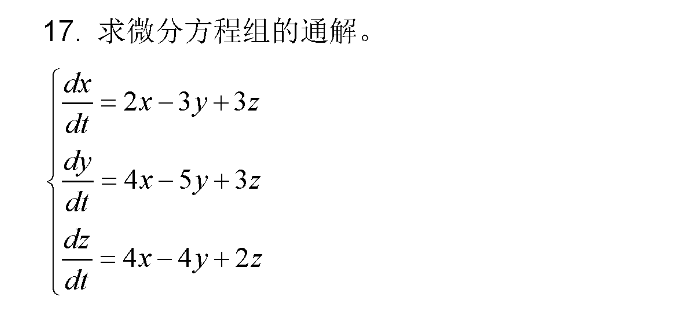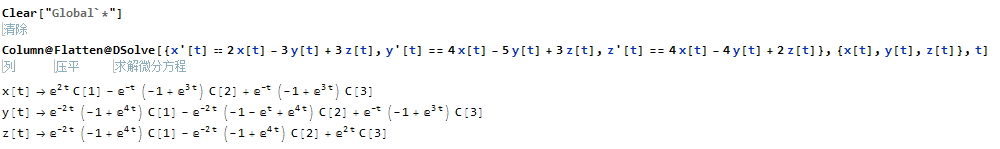Mathematica入门习题–微分方程组通解2017年10月4日20:48:2222 171字阅读0分34秒

第三十三题1. Clear["Global`*"]
2. Column@Flatten@
3. DSolve[{x'[t] == 2 x[t] - 3 y[t] + 3 z[t],
4. y'[t] == 4 x[t] - 5 y[t] + 3 z[t],
5. z'[t] == 4 x[t] - 4 y[t] + 2 z[t]}, {x[t], y[t], z[t]}, t]• 微信公众号
• 关注微信公众号
•• QQ群
• 我们的QQ群号
•• 本文由 发表于 2017年10月4日20:48:22
• 转载请务必保留本文链接：https://mathpretty.com/8197.html

•申城弛豫

为什么运行出来，显示错误呢？
sol = NDSolve[{x'[t] == 2 x[t] – 3 y[t] + 3 z[t],
y'[t] == 4 x[t] – 5 y[t] + 3 z[t],
z'[t] == 4 x[t] – 4 y[t] + 2 z[t]}, {x[t], y[t], z[t]}, t]

NDSolve::deqn: Equation or list of equations expected instead of True in the first argument {True,True,True}.
NDSolve[{True, True, True}, {x[t], y[t], z[t]}, t]

•王 茂南

@ 申城弛豫 首先使用NDSolve是需要规定t的范围的, 解通解可以使用DSolve. 其次好像只去直接复制会出现问题, 你可以尝试自己输入进去, 或者重新复制粘贴一下.